# Dr. Christopher BrandtFormer PhD Student at the Computer Graphics and Visualization group at TU Delft
E-mail: <C.Brandt_AT_tudelft.nl>

## Publications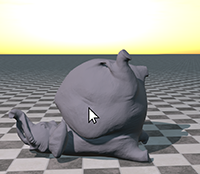Hyper-Reduced Projective Dynamics
Christopher Brandt, Elmar Eisemann, Klaus Hildebrandt
ACM Transactions on Graphics 37(4) Article 80 (SIGGRAPH 2018)
Abstract: We present a method for the real-time simulation of deformable objects that combines the robustness, generality, and high performance of Projective Dynamics with the efficiency and scalability offered by model reduction techniques. The method decouples the cost for time integration from the mesh resolution and can simulate large meshes in real-time. The proposed hyper-reduction of Projective Dynamics combines a novel fast approximation method for constraint projections and a scalable construction of sparse subspace bases. The resulting system achieves real-time rates for large subspaces enabling rich dynamics and can resolve general user interactions, collision constraints, external forces and changes to the materials. The construction of the hyper-reduced system does not require user-interaction and refrains from using training data or modal analysis, which results in a fast preprocessing stage.
[preprint][video][video (low res.)][supp. Material][demo][code][doi]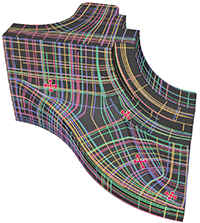Modeling n-Symmetry Vector Fields using Higher-Order Energies
Christopher Brandt, Leonardo Scandolo, Elmar Eisemann, Klaus Hildebrandt
ACM Transactions on Graphics 37(2) Article 18, 2018
To be presented at SIGGRAPH 2018
Abstract: We introduce a variational approach for modeling n-symmetry vector and direction fields on surfaces that supports interpolation and alignment constraints, placing singularities and local editing, while providing real-time responses. The approach is based on novel biharmonic and m-harmonic energies for n-fields on surface meshes and the integration of hard constraints to the resulting optimization problems. Real-time computation rates are achieved by a model reduction approach employing a Fourier-like n-vector field decomposition, which associates frequencies and modes to n-vector fields on surfaces. To demonstrate the benefits of the proposed n-field modeling approach, we use it for controlling stroke directions in line-art drawings of surfaces and for the modeling of anisotropic BRDFs which define the reflection behavior of surfaces.
[preprint][video][doi]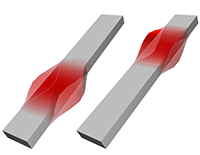Compressed Vibration Modes of Elastic Bodies
Christopher Brandt, Klaus Hildebrandt
Computer Aided Geometric Design 52–53 (Geometric Modeling and Processing 2017), pages 297–312
Abstract: The natural vibration modes of deformable objects are a fundamental physical phenomenon. In this paper, we introduce compressed vibration modes, which, in contrast to the natural vibration modes, are localized (“sparse”) deformations. The localization is achieved by augmenting the objective which has the vibration modes as minima by a L1 term. As a result the compressed modes form a compromise between localization and optimal energy efficiency of the deformations. We introduce a scheme for computing bases of compressed modes by solving sequences of convex optimization problems. Our experiments demonstrate that the resulting bases are well-suited for reduced-order shape deformation and for guiding the segmentation of objects into functional parts.
[preprint][video][doi]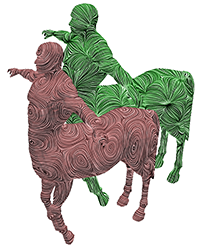Spectral Processing of Tangential Vector Fields
Christopher Brandt, Leonardo Scandolo, Elmar Eisemann, Klaus Hildebrandt
Computer Graphics Forum 36(6), pages 338–353, 2017
Presented at Eurographics 2017
Abstract: We propose a framework for the spectral processing of tangential vector fields on surfaces. The basis is a Fourier-type representation of tangential vector fields that associates frequencies with tangential vector fields. To implement the representation for piecewise constant tangential vector fields on triangle meshes, we introduce a discrete Hodge–Laplace operator that fits conceptually to the prominent cotan discretization of the Laplace–Beltrami operator. Based on the Fourier representation, we introduce schemes for spectral analysis, filtering and compression of tangential vector fields. Moreover, we introduce a spline-type editor for modeling of tangential vector fields with interpolation constraints for the field itself and its divergence and curl. Using the spectral representation, we propose a numerical scheme that allows for real-time modeling of tangential vector fields.
[preprint][video][supp. material][doi]Geometric Flows of Curves in Shape Space for Processing Motion of Deformable Objects
Christopher Brandt, Christoph von Tycowicz, Klaus Hildebrandt
Computer Graphics Forum 35(2) (Eurographics 2016), pages 295–305
Best Paper Honorable Mention Award at Eurographics 2016
Abstract: We introduce techniques for the processing of motion and animations of non-rigid shapes. The idea is to regard animations of deformable objects as curves in shape space. Then, we use the geometric structure on shape space to transfer concepts from curve processing in Rn to the processing of motion of non-rigid shapes. Following this principle, we introduce a discrete geometric flow for curves in shape space. The flow iteratively replaces every shape with a weighted average shape of a local neighborhood and thereby globally decreases an energy whose minimizers are discrete geodesics in shape space. Based on the flow, we devise a novel smoothing filter for motions and animations of deformable shapes. By shortening the length in shape space of an animation, it systematically regularizes the deformations between consecutive frames of the animation. The scheme can be used for smoothing and noise removal, e.g., for reducing jittering artifacts in motion capture data. We introduce a reduced-order method for the computation of the flow. In addition to being efficient for the smoothing of curves, it is a novel scheme for computing geodesics in shape space. We use the scheme to construct non-linear Bézier curves by executing de Casteljau’s algorithm in shape space.
[preprint][video][doi]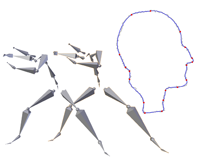Optimal Spline Approximation via l0-Minimization
Christopher Brandt, Hans-Peter Seidel, Klaus Hildebrandt
Computer Graphics Forum 34(2) (Eurographics 2015), pages 617–626.
Abstract: Splines are part of the standard toolbox for the approximation of functions and curves in Rd. Still, the problem of finding the spline that best approximates an input function or curve is ill-posed, since in general this yields a “spline” with an infinite number of segments. The problem can be regularized by adding a penalty term for the number of spline segments. We show how this idea can be formulated as an l0-regularized quadratic problem. This gives us a notion of optimal approximating splines that depends on one parameter, which weights the approximation error against the number of segments. We detail this concept for different types of splines including B-splines and composite Bézier curves. Based on the latest development in the field of sparse approximation, we devise a solver for the resulting minimization problems and show applications to spline approximation of planar and space curves and spline conversion of motion capture data.
[preprint][video][doi]

## Co-authored: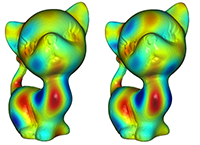Fast Approximation of Laplace–Beltrami Eigenproblems Ahmad Nasikun, Christopher Brandt, Klaus Hildebrandt Computer Graphics Forum 37(5) (Symposium on Geometry Processing 2018), pages 121–134 Abstract: The spectrum and eigenfunctions of the Laplace–Beltrami operator are at the heart of effective schemes for a variety of problems in geometry processing. A burden attached to these spectral methods is that they need to numerically solve a large-scale eigenvalue problem, which results in costly precomputation. In this paper, we address this problem by proposing a fast approximation algorithm for the lowest part of the spectrum of the Laplace–Beltrami operator. Our experiments indicate that the resulting spectra well-approximate reference spectra, which are computed with state-of-the-art eigensolvers. Moreover, we demonstrate that for different applications comparable results are produced with the approximate and the reference spectra and eigenfunctions. The benefits of the proposed algorithm are that the cost for computing the approximate spectra is just a fraction of the cost required for numerically solving the eigenvalue problems, the storage requirements are reduced and evaluation times are lower. Our approach can help to substantially reduce the computational burden attached to spectral methods for geometry processing.  [preprint][supp. document][video 1][video 2][doi]